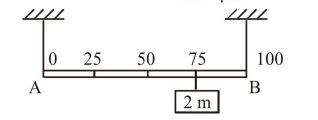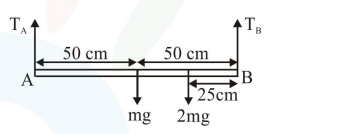# Solve this following

Question:+

Shown in the figure is rigid and uniform one meter long rod $\mathrm{AB}$ held in horizontal position by two strings tied to its ends and attached to the ceiling. The rod is of mass ' $m$ ' and has another weight of mass $2 \mathrm{~m}$ hung at a distance of $75 \mathrm{~cm}$ from A. The tension in the string at $\mathrm{A}$ is :

1. $2 \mathrm{mg}$

2. $0.5 \mathrm{mg}$

3. $0.75 \mathrm{mg}$

4. $1 \mathrm{mg}$

Correct Option: , 4

Solution:$\tau_{\mathrm{B}}=0$ (torque about point $\mathrm{B}$ is zero)

$\left(\mathrm{T}_{\mathrm{A}}\right) \times 100-(\mathrm{mg}) \times 50-(2 \mathrm{mg}) \times 25=0$

$100 \mathrm{~T}_{\mathrm{A}}=100 \mathrm{mg}$

$\mathrm{T}_{\mathrm{A}}=1 \mathrm{mg}$